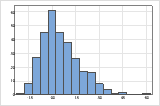# Interpret the key results for Paired t

Complete the following steps to interpret a paired t-test. Key output includes the estimate of the mean of the difference, the confidence interval, the p-value, and several graphs.

## Step 1: Determine a confidence interval for the population mean difference

First, consider the mean difference, and then examine the confidence interval. The mean difference is the average of the differences between the paired observations in your sample.

The mean difference is an estimate of the population mean difference. Because the mean difference is based on sample data and not on the entire population, it is unlikely that the sample mean difference equals the population mean difference. To better estimate the population mean difference, use the confidence interval of the difference.

The confidence interval provides a range of likely values for the population mean difference of the paired observations. For example, a 95% confidence level indicates that if you take 100 random samples from the population, you could expect approximately 95 of the samples to produce intervals that contain the population mean difference. The confidence interval helps you assess the practical significance of your results. Use your specialized knowledge to determine whether the confidence interval includes values that have practical significance for your situation. If the interval is too wide to be useful, consider increasing your sample size. For more information, go to Ways to get a more precise confidence interval.

## Step 2: Determine whether the test results are statistically significant

To determine whether the difference between the population means is statistically significant, compare the p-value to the significance level. Usually, a significance level (denoted as α or alpha) of 0.05 works well. A significance level of 0.05 indicates a 5% risk of concluding that a difference exists when there is no actual difference.
P-value ≤ α: The difference between the means is statistically significant (Reject H0)
If the p-value is less than or equal to the significance level, the decision is to reject the null hypothesis. You can conclude that the difference between the population means does not equal the hypothesized difference. If you did not specify a hypothesized difference, Minitab tests whether there is no difference between the means (Hypothesized difference = 0) Use your specialized knowledge to determine whether the difference is practically significant. For more information, go to Statistical and practical significance.
P-value > α: The difference between the means is not statistically significant (Fail to reject H0)
If the p-value is greater than the significance level, the decision is to fail to reject the null hypothesis. You do not have enough evidence to conclude that the mean difference between the paired observations is statistically significant. You should make sure that your test has enough power to detect a difference that is practically significant. For more information, go to Power and Sample Size for Paired t.

## Step 3: Check your data for problems

Problems with your data, such as skewness and outliers, can adversely affect your results. Use graphs to look for skewness and to identify potential outliers.

When data are skewed, the majority of the data are located on the high or low side of the graph. Often, skewness is easiest to detect with a histogram or boxplot.The histogram with right-skewed data shows wait times. Most of the wait times are relatively short, and only a few wait times are long. The histogram with left-skewed data shows failure time data. A few items fail immediately, and many more items fail later.

Data that are severely skewed can affect the validity of the p-value if your sample is small (less than 20 values). If your data are severely skewed and you have a small sample, consider increasing your sample size.In this histogram, the data do not appear to be severely skewed.

### Identify outliers

Outliers, which are data values that are far away from other data values, can strongly affect the results of your analysis. Often, outliers are easiest to identify on a boxplot.